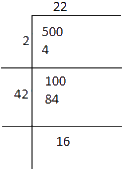# Ex.6.4 Q9 Squares and Square Roots - NCERT Maths Class 8

Go back to  'Ex.6.4'

## Question

There are $$500$$ children in a school. For a P.T. drill they have to stand in such a manner that the number of rows is equal to number of columns. How many children would be left out in this arrangement?

Here, we have to find the number which should be subtracted from total number of children to make it a perfect square.

Video Solution
Squares And Square Roots
Ex 6.4 | Question 9

## Text Solution

What is known?

Number of children in a school.

What is unknown?

Number of children would be left out in PT drill.

Reasoning:

Here, we have to find the number which should be subtracted from total number of children to make it a prefect square.

Steps:

Number of children in a school $$= 500$$ The square root of $$500$$ can be calculated using long division method.The remainder is $$16$$. It shows that $${(22)^2}$$ is less than $$500$$.

Therefore, perfect square can be obtained by subtracting $$16$$ from the given number.

Therefore, required perfect square is

\begin{align}&= 500 - 16\\&= 484\end{align}

$${\rm{And}}\;\sqrt {484}= 22$$

Number of children left out in PT drill arrangement $$= 16$$

Learn from the best math teachers and top your exams

• Live one on one classroom and doubt clearing
• Practice worksheets in and after class for conceptual clarity
• Personalized curriculum to keep up with school